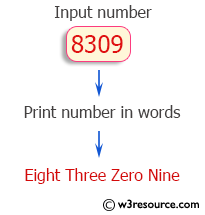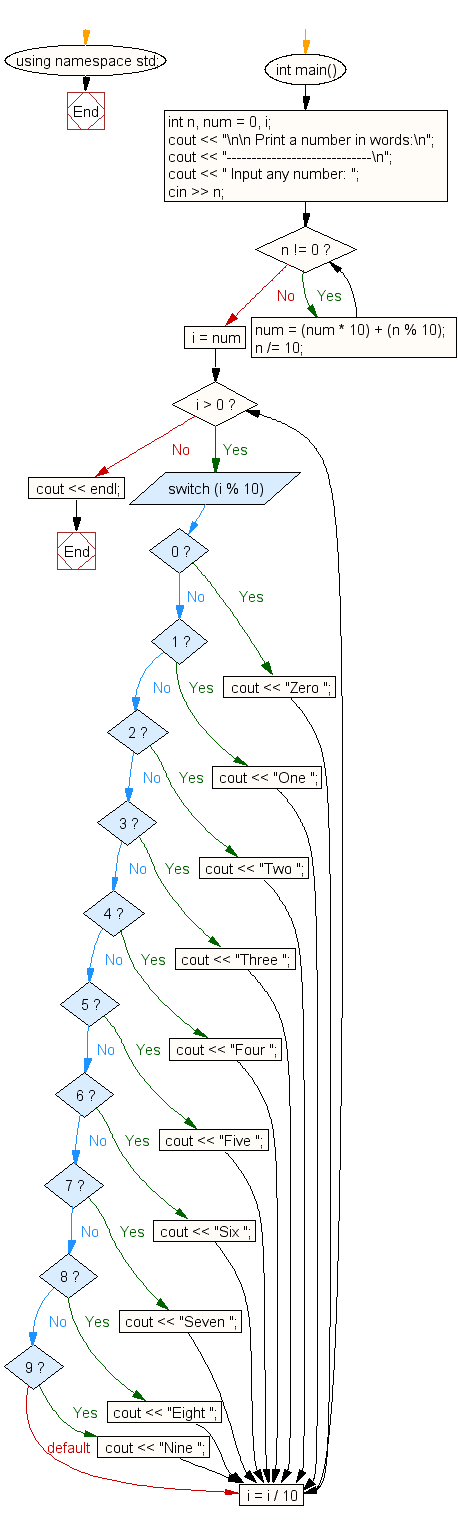﻿ C++ Exercises: Input any number and print it in words - w3resource

# C++ Exercises: Input any number and print it in words

## C++ For Loop: Exercise-60 with Solution

Write a program in C++ to input any number and print it in words.

Pictorial Presentation:Sample Solution:-

C++ Code :

``````#include <iostream>
using namespace std;
int main()
{
int n, num = 0, i;
cout << "\n\n Print a number in words:\n";
cout << "-----------------------------\n";
cout << " Input any number: ";
cin >> n;
while (n != 0) {
num = (num * 10) + (n % 10);
n /= 10;
}
for (i = num; i > 0; i = i / 10) {

switch (i % 10) {
case 0:
cout << "Zero ";
break;
case 1:
cout << "One ";
break;
case 2:
cout << "Two ";
break;
case 3:
cout << "Three ";
break;
case 4:
cout << "Four ";
break;
case 5:
cout << "Five ";
break;
case 6:
cout << "Six ";
break;
case 7:
cout << "Seven ";
break;
case 8:
cout << "Eight ";
break;
case 9:
cout << "Nine ";
break;
}
}
cout << endl;
}
``````

Sample Output:

``` Print a number in words:
-----------------------------
Input any number: 8309
Eight Three Zero Nine
```

Flowchart:C++ Code Editor: